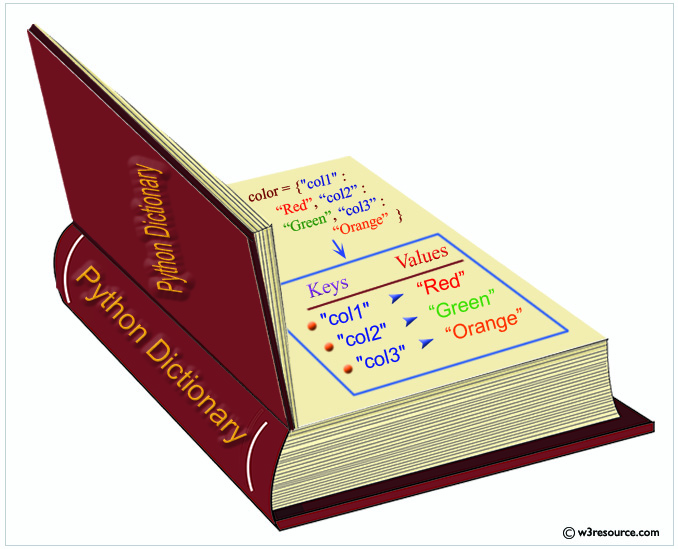# Python Dictionary

## Dictionary

Python dictionary is a container of the unordered set of objects like lists. The objects are surrounded by curly braces { }. The items in a dictionary are a comma-separated list of key:value pairs where keys and values are Python data type.

Each object or value accessed by key and keys are unique in the dictionary. As keys are used for indexing, they must be the immutable type (string, number, or tuple). You can create an empty dictionary using empty curly braces.Dictionary: Commands

```# Coll. of keys that reflects changes.
<view> = <dict>.keys()
# Coll. of values that reflects changes.
<view> = <dict>.values()
# Coll. of key-value tuples.
<view> = <dict>.items()
# Returns default if key is missing.
value  = <dict>.get(key, default=None)
# Returns and writes default if key is missing.
value  = <dict>.setdefault(key, default=None)
# Creates a dict with default value of type.
<dict> = collections.defaultdict(<type>)
# Creates a dict with default value 1.
<dict> = collections.defaultdict(lambda: 1)
<dict>.update(<dict>)
# Creates a dict from coll. of key-value pairs.
<dict> = dict(<collection>)
# Creates a dict from two collections.
<dict> = dict(zip(keys, values))
# Creates a dict from collection of keys.
<dict> = dict.fromkeys(keys [, value])
# Removes item or raises KeyError.
value = <dict>.pop(key)
# Filters dictionary by keys.
{k: v for k, v in <dict>.items() if k in keys}
Counter
>>> from collections import Counter
>>> colors = ['blue', 'red', 'blue', 'red', 'blue']
>>> counter = Counter(colors)
>>> counter['yellow'] += 1
Counter({'blue': 3, 'red': 2, 'yellow': 1})
>>> counter.most_common()
('blue', 3)
```

Create a new dictionary in Python

``````>>> #Empty dictionary
>>> new_dict = dict()
>>> new_dict = {}
>>> print(new_dict)
{}
>>> #Dictionary with key-vlaue
>>> color = {"col1" : "Red", "col2" : "Green", "col3" : "Orange" }
>>> color
{'col2': 'Green', 'col3': 'Orange', 'col1': 'Red'}
>>>
``````

Get value by key in Python dictionary

``````>>> #Declaring a dictionary
>>> dict = {1:20.5, 2:3.03, 3:23.22, 4:33.12}
>>> #Access value using key
>>> dict
20.5
>>> dict
23.22
>>> #Accessing value using get() method
>>> dict.get(1)
20.5
>>> dict.get(3)
23.22
>>>
``````

Add key/value to a dictionary in Python

``````>>> #Declaring a dictionary with a single element
>>> dic = {'pdy1':'DICTIONARY'}
>>>print(dic)
{'pdy1': 'DICTIONARY'}
>>> dic['pdy2'] = 'STRING'
>>> print(dic)
{'pdy1': 'DICTIONARY', 'pdy2': 'STRING'}
>>>
>>> #Using update() method to add key-values pairs in to dictionary
>>> d = {0:10, 1:20}
>>> print(d)
{0: 10, 1: 20}
>>> d.update({2:30})
>>> print(d)
{0: 10, 1: 20, 2: 30}
>>>
``````

Iterate over Python dictionaries using for loops

Code:

``````d = {'Red': 1, 'Green': 2, 'Blue': 3}
for color_key, value in d.items():
print(color_key, 'corresponds to ', d[color_key])
``````

Output:

```>>>
Green corresponds to  2
Red corresponds to  1
Blue corresponds to  3
>>>
```

Remove a key from a Python dictionary

Code:

``````myDict = {'a':1,'b':2,'c':3,'d':4}
print(myDict)
if 'a' in myDict:
del myDict['a']
print(myDict)
``````

Output:

```>>>
{'d': 4, 'a': 1, 'b': 2, 'c': 3}
{'d': 4, 'b': 2, 'c': 3}
>>>
```

Sort a Python dictionary by key

Code:

``````color_dict = {'red':'#FF0000',
'green':'#008000',
'black':'#000000',
'white':'#FFFFFF'}

for key in sorted(color_dict):
print("%s: %s" % (key, color_dict[key]))
``````

Output:

```>>>
black: #000000
green: #008000
red: #FF0000
white: #FFFFFF
>>>
```

Find the maximum and minimum value of a Python dictionary

Code:

``````my_dict = {'x':500, 'y':5874, 'z': 560}

key_max = max(my_dict.keys(), key=(lambda k: my_dict[k]))
key_min = min(my_dict.keys(), key=(lambda k: my_dict[k]))

print('Maximum Value: ',my_dict[key_max])
print('Minimum Value: ',my_dict[key_min])
``````

Output:

```>>>
Maximum Value:  5874
Minimum Value:  500
>>>
```

Concatenate two Python dictionaries into a new one

Code:

``````dic1={1:10, 2:20}
dic2={3:30, 4:40}
dic3={5:50,6:60}
dic4 = {}
for d in (dic1, dic2, dic3): dic4.update(d)
print(dic4)
``````

Output:

```>>>
{1: 10, 2: 20, 3: 30, 4: 40, 5: 50, 6: 60}
>>>
```

Test whether a Python dictionary contains a specific key

Code:

``````fruits = {}
fruits["apple"] = 1
fruits["mango"] = 2
fruits["banana"] = 4

# Use in.
if "mango" in fruits:
print("Has mango")
else:
print("No mango")

# Use in on nonexistent key.
if "orange" in fruits:
print("Has orange")
else:
print("No orange")
``````

Output

```>>>
Has mango
No orange
>>>
```

Find the length of a Python dictionary

Code:

``````fruits = {"mango": 2, "orange": 6}

# Use len() function to get the length of the dictionary
print("Length:", len(fruits))
``````

Output:

```>>>
Length: 2
>>>```

Previous: Python Lists
Next: Python Tuples

Test your Python skills with w3resource's quiz

﻿

## Python: Tips of the Day

Clamps num within the inclusive range specified by the boundary values x and y:

Example:

```def tips_clamp_num(num,x,y):
return max(min(num, max(x, y)), min(x, y))
print(tips_clamp_num(2, 4, 6))
print(tips_clamp_num(1, -1, -6))
```

Output:

```4
-1
```